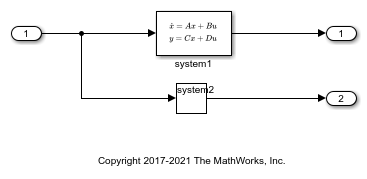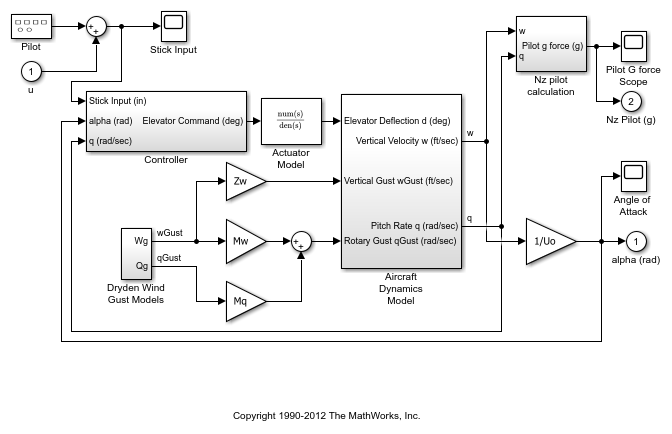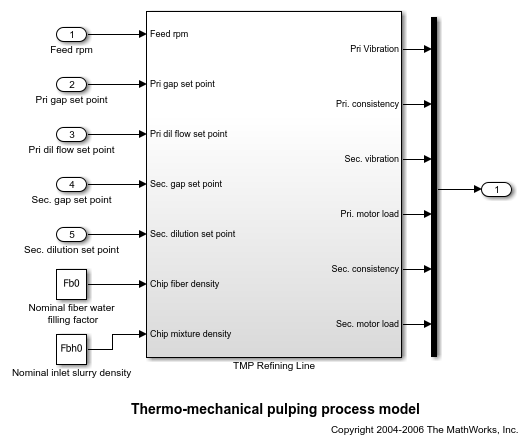# getOutputIndex

Get index of an output element of an operating point specification

## Syntax

``index = getOutputIndex(op,block)``
``index = getOutputIndex(op,block,port)``
``index = getOutputIndex(op,block,port,element)``

## Description

The `Outputs` property of an operating point specification is an array that contains trimming specifications for each model output. When defining a mapping function for customized trimming of Simulink® models, you can use `getOutputIndex` to obtain the index of an output specification based on the corresponding block path.

When trimming Simulink models using optimization-based search, some applications require additional flexibility in defining the optimization search parameters. For such systems, you can specify custom constraints and a custom objective function. For complex models, you can define a mapping that selects a subset of the model states, inputs, and outputs to pass to the custom constraint and objective functions. For more information, see Compute Operating Points Using Custom Constraints and Objective Functions.

example

````index = getOutputIndex(op,block)` returns the index of the output specification that corresponds to `block` in the `Outputs` property of operating point specification `op`.```

example

````index = getOutputIndex(op,block,port)` returns the index of the output specification that corresponds to the trim output constraint added to the specified output `port` of the specified `block`.Use this syntax when the `Outputs` property of `op` contains trim output constraints for more than one signal originating from the same block.```

example

````index = getOutputIndex(op,block,port,element)` returns the index of the specified `element` within an output specification for an output with multiple elements.```

## Examples

collapse all

```mdl = 'scdindex1'; open_system(mdl) ```Create an operating point specification for model.

```opspec = operspec(mdl); ```

`opspec` contains an array of output specifications for the model.

```opspec.Outputs ```
```ans = y Known Min Max _____ _____ _____ _____ (1.) scdindex1/Out1 0 false -Inf Inf (2.) scdindex1/Out2 0 false -Inf Inf ```

Get the index of the output specification for Out2.

```idx = getOutputIndex(opspec,'scdindex1/Out2') ```
```idx = 2 1 ```

The first column of `idx` contains the index of the output specification in `opspec.Outputs`. The second column contains the element index within the output specification. In this case, there is only one element in the output specification.

```mdl = 'scdplane'; open_system(mdl) ```Create an operating point specification for the model.

```opspec = operspec(mdl); ```

In addition to root-level outputs of a model, the `opspec.Outputs` array contains specifications for trim constraints added to signals using the `addoutputspec` command.

Add an output specification to the signal originating from second output port of the Aircraft Dynamics Model block.

```opspec = addoutputspec(opspec,'scdplane/Aircraft Dynamics Model',2); ```

View the output array of `opspec`.

```opspec.Outputs ```
```ans = y Known Min Max _____ _____ _____ _____ (1.) scdplane/alpha (rad) 0 false -Inf Inf (2.) scdplane/Nz Pilot (g) 0 false -Inf Inf (3.) scdplane/Aircraft Dynamics Model 0 false -Inf Inf ```

Get the index of the added output specification. When there is an output specification for only one of the output ports of a given block, you do not need to specify the port number to get the output index.

```index1 = getOutputIndex(opspec,'scdplane/Aircraft Dynamics Model') ```
```index1 = 3 1 ```

Add an output specification to the signal originating from the first output of the same block.

```opspec = addoutputspec(opspec,'scdplane/Aircraft Dynamics Model',1); ```

View the output array of `opspec`.

```opspec.Outputs ```
```ans = y Known Min Max _____ _____ _____ _____ (1.) scdplane/alpha (rad) 0 false -Inf Inf (2.) scdplane/Nz Pilot (g) 0 false -Inf Inf (3.) scdplane/Aircraft Dynamics Model 0 false -Inf Inf (4.) scdplane/Aircraft Dynamics Model 0 false -Inf Inf ```

There are now two output specifications that correspond to the same block, one for each output port. Obtain the index for the output specification that corresponds with the output port `1` of the Aircraft Dynamics Model block.

```index2 = getOutputIndex(opspec,'scdplane/Aircraft Dynamics Model',1) ```
```index2 = 4 1 ```

```mdl = 'scdtmp'; open_system(mdl) ```Create an operating point specification object for the model.

```opspec = operspec(mdl); ```

`opspec` contains an output specification for the output port Out1, which is a vector signal.

```opspec.Outputs ```
```ans = y Known Min Max _____ _____ _____ _____ (1.) scdtmp/Out1 0 false -Inf Inf 0 false -Inf Inf 0 false -Inf Inf 0 false -Inf Inf 0 false -Inf Inf 0 false -Inf Inf ```

Obtain the indices of all the elements of Out1.

```index1 = getOutputIndex(opspec,'scdtmp/Out1') ```
```index1 = 1 1 1 2 1 3 1 4 1 5 1 6 ```

Each row of `index1` contains the index for one element of the vector signal in Out1. The first column is the index of the output specification object for the Out1 port in the `opsepc.Outputs`. The second column is the element index within the output specification.

You can also obtain the index for individual elements of an output specification, or a subset of elements. Get the index of element number `4` of Out1.

```index2 = getOutputIndex(opspec,'scdtmp/Out1',[],4) ```
```index2 = 1 4 ```

Get the indices of elements `2` and `3` of Out1.

```index3 = getOutputIndex(opspec,'scdtmp/Out1',[],[2 3]) ```
```index3 = 1 2 1 3 ```

## Input Arguments

collapse all

Operating point specification for a Simulink model, specified as an `OperatingSpec` or `OperatingReport`.

Block path that corresponds to an output specification in the `Outputs` property of `op`, specified as a character vector or string that contains the path of one of the following:

• Root-level output of the model.

• Source block for a signal in the model to which an output specification has been added. For more information on adding output specifications to a model, see `addoutputspec`.

To see all the blocks that have output specifications, view the `Outputs` property of `op`.

`op.Outputs`

Output port, specified as an integer in the range `[1,N]`, where `N` is the number of output ports on the specified `block`. If `block` is a root-level output port, then `N` is `1`.

If you do not specify `port`, and there is one entry in the output array of `op` that corresponds to the specified `block`, then the default value of `port` is the port number of that entry. If there are multiple entries in the output array that correspond to the specified `block`, then the default value of `port` is the port number of the first entry. For an example, see Get Index of Trim Output Specification Added To Signal.

To view the port number of the `i`th entry in the output array of `op`, type:

`op.Outputs(i).PortNumber`

Output element index, specified as a positive integer less than or equal to the port width of the output of the specified `block`, or a vector of such integers. By default, if you do not specify `element`, `getOutputIndex` returns the indices of all elements in the selected output specification. For an example, see Get Output Indices for Output Specification with Multiple Elements.

## Output Arguments

collapse all

Output index, returned as a 2-element row vector when `element` is an integer, or a 2-column array when `element` is a vector. Each row of `index` contains the index for a single output element.

The first column of `index` contains the index of the corresponding output specification in the `Outputs` property of `op`. The second column contains the element index within the output specification.

Using `index`, you can specify the output portion of a custom mapping for customized trimming of Simulink models. For more information, see the `CustomMappingFcn` property of `operspec`.

## Version History

Introduced in R2017a